Ex 6.1

Chapter 6 Class 7 Triangle and its Properties
Serial order wise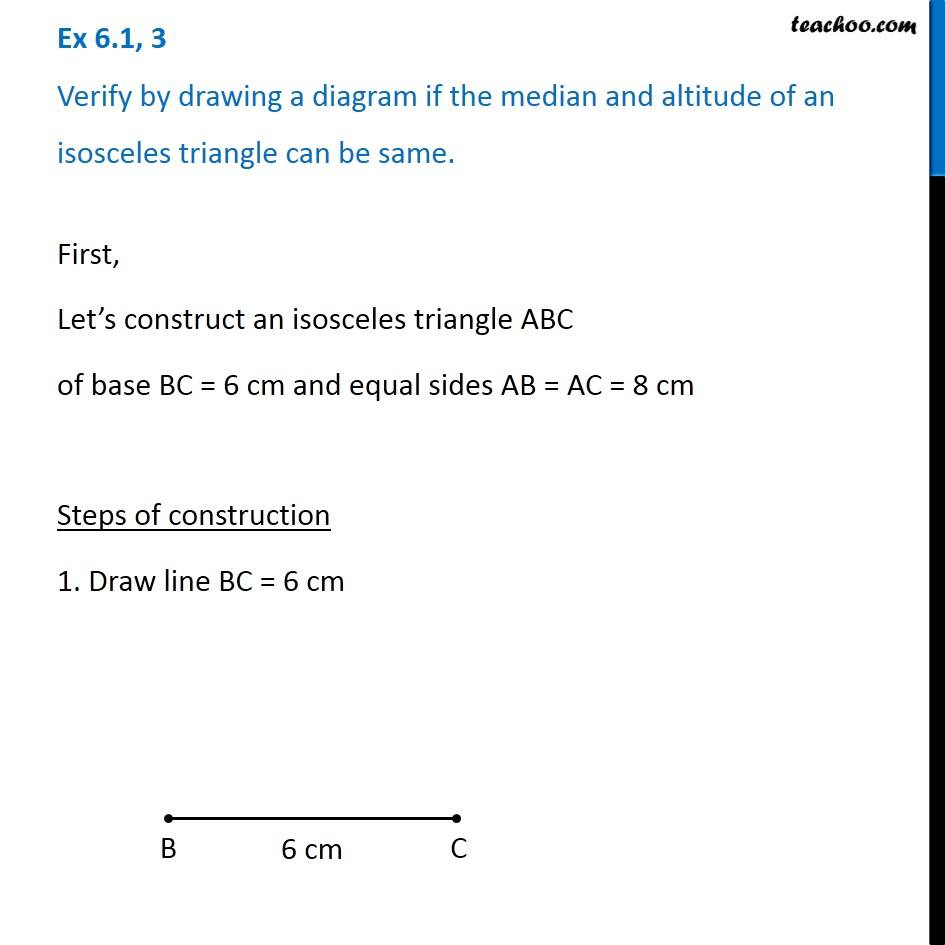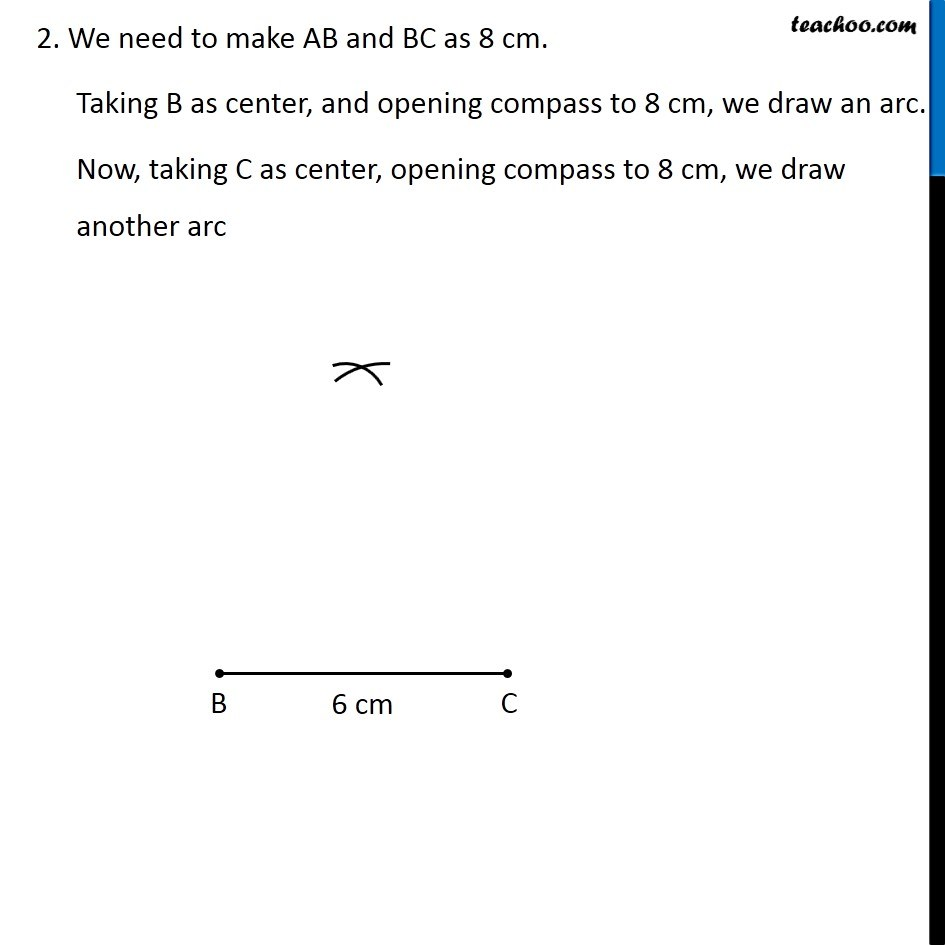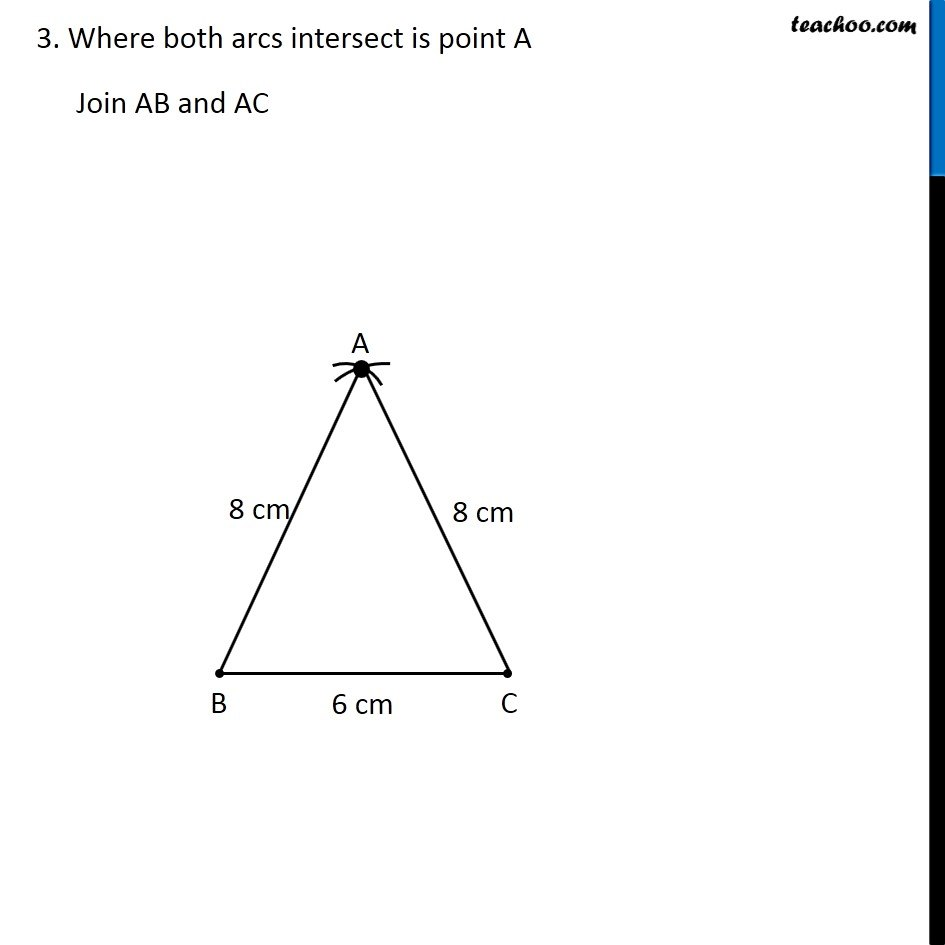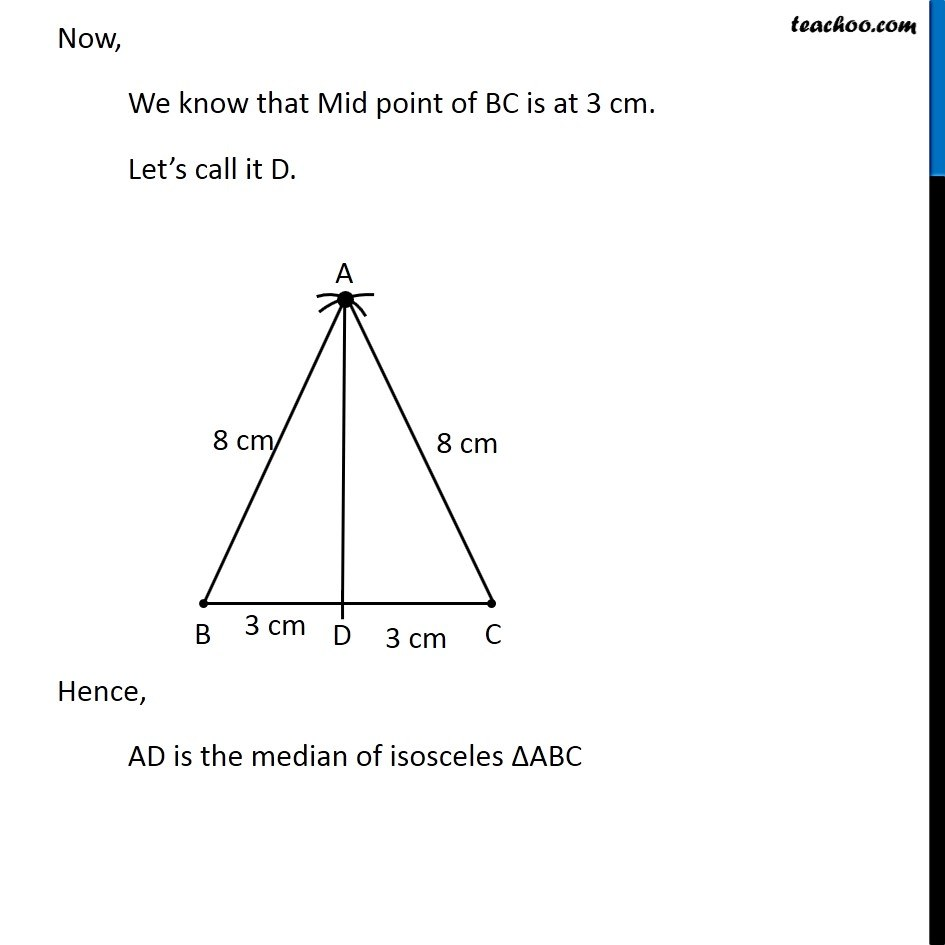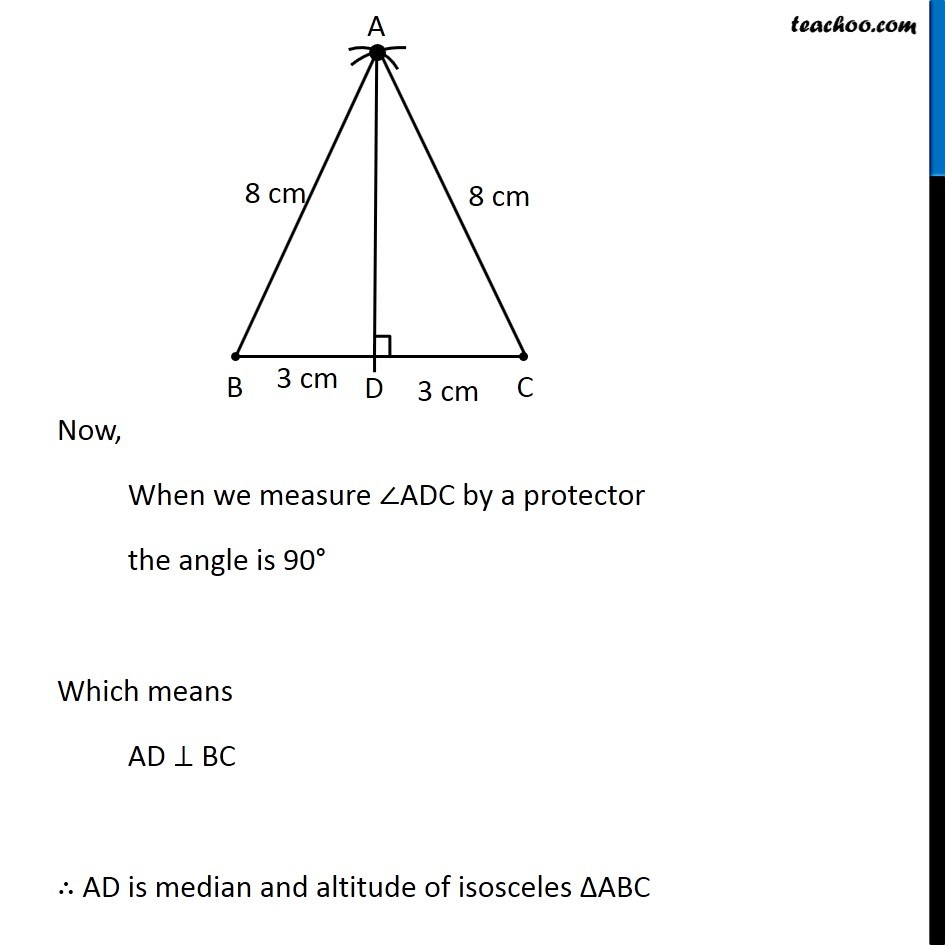Learn in your speed, with individual attention - Teachoo Maths 1-on-1 Class

### Transcript

Ex 6.1, 3 Verify by drawing a diagram if the median and altitude of an isosceles triangle can be same.First, Let’s construct an isosceles triangle ABC of base BC = 6 cm and equal sides AB = AC = 8 cm Steps of construction 1. Draw line BC = 6 cm 2. We need to make AB and BC as 8 cm. Taking B as center, and opening compass to 8 cm, we draw an arc. Now, taking C as center, opening compass to 8 cm, we draw another arc 3. Where both arcs intersect is point A Join AB and AC Now, We know that Mid point of BC is at 3 cm. Let’s call it D. Hence, AD is the median of isosceles ∆ABC Now, When we measure ∠ADC by a protector the angle is 90° Which means AD ⊥ BC ∴ AD is median and altitude of isosceles ∆ABC